Updating search results...

# 54 Results

View
Selected filters:
• NC.Math.3.NF.2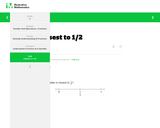Rating
0.0 stars

This instructional task asks students to determine which number is closest to 1/2. Students must use problem-solving skills to help them complete the task.

Subject:
Mathematics
Material Type:
Activity/Lab
Provider:
Illustrative Mathematics
Author:
Illustrative Mathematics
02/26/2019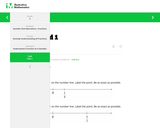Rating
0.0 stars

For this instructional task, students are given 2 number lines and are asked to locate the number 1 on each, being as exact as possible.

Subject:
Mathematics
Material Type:
Activity/Lab
Provider:
Illustrative Mathematics
Author:
Illustrative Mathematics
02/26/2019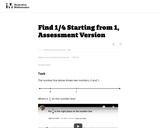Unrestricted Use
CC BY
Rating
0.0 stars

This is a task from the Illustrative Mathematics website that is one part of a complete illustration of the standard to which it is aligned. Each task has at least one solution and some commentary that addresses important asects of the task and its potential use. Here are the first few lines of the commentary for this task: The number line below shows two numbers, 0 and 1. Where is $\frac14$ on this number line? http://www.youtube.com/watch?v=HJ0LDgxVGfU...

Subject:
Mathematics
Material Type:
Activity/Lab
Provider:
Illustrative Mathematics
Provider Set:
Illustrative Mathematics
Author:
Illustrative Mathematics
03/18/2013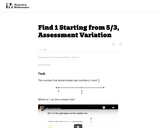Unrestricted Use
CC BY
Rating
0.0 stars

This is a task from the Illustrative Mathematics website that is one part of a complete illustration of the standard to which it is aligned. Each task has at least one solution and some commentary that addresses important asects of the task and its potential use. Here are the first few lines of the commentary for this task: The number line below shows two numbers, 0 and $\frac53$. Where is 1 on this number line? http://www.youtube.com/watch?v=wAXRjMUgiu4...

Subject:
Mathematics
Material Type:
Activity/Lab
Provider:
Illustrative Mathematics
Provider Set:
Illustrative Mathematics
Author:
Illustrative Mathematics
03/18/2013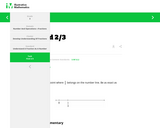Rating
0.0 stars

For this instructional task, students label the point where 2/3 belongs on the number line, being as exact as possible.

Subject:
Mathematics
Material Type:
Activity/Lab
Provider:
Illustrative Mathematics
Author:
Illustrative Mathematics
02/26/2019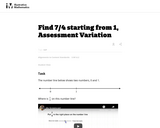Unrestricted Use
CC BY
Rating
0.0 stars

This is a task from the Illustrative Mathematics website that is one part of a complete illustration of the standard to which it is aligned. Each task has at least one solution and some commentary that addresses important asects of the task and its potential use. Here are the first few lines of the commentary for this task: The number line below shows two numbers, 0 and 1. Where is $\frac74$ on this number line? http://www.youtube.com/watch?v=TEzH_PbHZIw...

Subject:
Mathematics
Material Type:
Activity/Lab
Provider:
Illustrative Mathematics
Provider Set:
Illustrative Mathematics
Author:
Illustrative Mathematics
03/18/2013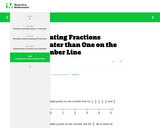Rating
0.0 stars

For this instructional task, students mark and label points on the number line.

Subject:
Mathematics
Material Type:
Activity/Lab
Provider:
Illustrative Mathematics
Author:
Illustrative Mathematics
02/26/2019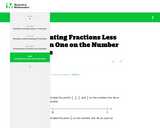Rating
0.0 stars

For this instructional task, students mark and label points on the number line.

Subject:
Mathematics
Material Type:
Activity/Lab
Provider:
Illustrative Mathematics
Author:
Illustrative Mathematics
02/26/2019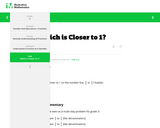Rating
0.0 stars

For this instructional task, students identify the fraction that is closer to 1 on a number line.

Subject:
Mathematics
Material Type:
Activity/Lab
Provider:
Illustrative Mathematics
Author:
Illustrative Mathematics
02/26/2019Conditional Remix & Share Permitted
CC BY-NC-SA
Rating
0.0 stars

This parent guide supports parents in helping their child at home with the 3rd grade Math content.

Subject:
Mathematics
Material Type:
Reference Material
Vocabulary
Author:
AMBER GARVEY
02/13/2023Conditional Remix & Share Permitted
CC BY-NC-SA
Rating
0.0 stars

This resource accompanies our Rethink 3rd Grade Math Course. It includes ideas for use, ways to support exceptional children, ways to extend learning, digital resources and tools, tips for supporting English Language Learners and students with visual and hearing impairments. There are also ideas for offline learning.

Subject:
Mathematics
Material Type:
Curriculum
Lesson Plan
Teaching/Learning Strategy
Author:
AMBER GARVEY
03/14/2023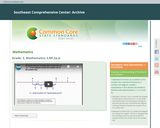Rating
0.0 stars

The Common Core State Standards (CCSS) videos are designed to support states, schools, and teachers in the implementation of selected math standards. Each video is an audiovisual resource that focuses on one or more specific standards through examples and illustrations geared to enhancing understanding. The intent of each content-focused video is to clarify the meaning of the individual standard rather than to be a guide on how to teach each standard, although the examples can be adapted for instructional use.

Subject:
Mathematics
Material Type:
Lesson
Provider:
The Southeast Comprehensive Center
Author:
The Southeast Comprehensive Center
02/26/2019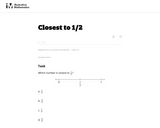Unrestricted Use
CC BY
Rating
0.0 stars

In this task using a number line, students must partition the interval between 0 and 1 into eighths.

Subject:
Mathematics
Material Type:
Activity/Lab
Provider:
Illustrative Mathematics
Provider Set:
Illustrative Mathematics
Author:
Illustrative Mathematics
05/01/2012Unrestricted Use
CC BY
Rating
0.0 stars

In this lesson students practice fractions by sharing certain numbers of objects among different numbers of people. They begin with unit fractions and move to sharing multiple objects among multiple people, in which a < b, and to produce fractions less than one.

Subject:
Mathematics
Material Type:
Activity/Lab
Provider:
PBS
Author:
WNET
04/04/2008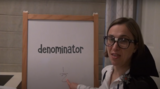Unrestricted Use
CC BY
Rating
0.0 stars

This lesson is Day 10 in a series of 12 lessons around fraction equivalences and comparisons.

This video lesson serves as a review of equpartitioning in order to determine the fractional part of an area or length. This is a necessary foundational skill for comparing and finding equivalent fractions.

Subject:
Mathematics
Material Type:
Activity/Lab
Interactive
Lesson
Simulation
Author:
Dawne Coker
06/25/2020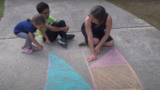Unrestricted Use
CC BY
Rating
0.0 stars

This lesson is Day 11 in a series of 12 lessons around fraction equivalences and comparisons.

This video lesson reviews parts of a whole, comparing fractions, and equivalent fractions. No materials are needed.

Subject:
Mathematics
Material Type:
Activity/Lab
Interactive
Lesson
Simulation
Author:
Dawne Coker
06/25/2020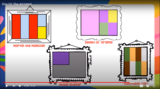Unrestricted Use
CC BY
Rating
0.0 stars

This lesson is Day 12 in a series of 12 lessons around fraction equivalences and comparisons.

Use your math skills to help detectives solve the crime. This video lesson reviews fractions of an area, fractions on a number line, and equivalent fractions. No materials are required.

Subject:
Mathematics
Material Type:
Activity/Lab
Interactive
Lesson
Simulation
Author:
Dawne Coker
06/25/2020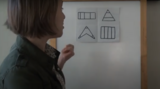Unrestricted Use
Public Domain
Rating
0.0 stars

This lesson is Day 1 in a series of 12 lessons around fraction equivalences and comparisons.

This video lesson encourages students to reason about and visualize fractions. The lesson focuses on the ideas that fractions are fair shares and that fractions can be compared. This lesson assumes that students have some initial experiences with fractions.

Subject:
Mathematics
Material Type:
Interactive
Lesson
Presentation
Simulation
Author:
Dawne Coker
06/25/2020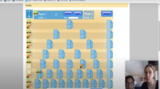Unrestricted Use
CC BY
Rating
0.0 stars

This lesson is Day 2 in a series of 12 lessons around fraction equivalences and comparisons.

This game may be played with one or two people. It reviews fractions on a number line, adding fraction with like denominators, and equivalent fractions. For an extra challenge, you can even break up your moves across multiple tracks (e.g., if your card reads 1/2, you could move 1/4 and 2/8).

https://www.nctm.org/Classroom-Resources/Illuminations/Interactives/Playing-Fraction-Track/

Subject:
Mathematics
Material Type:
Activity/Lab
Interactive
Lesson
Simulation
Author:
Dawne Coker
06/25/2020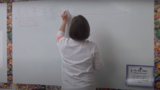Unrestricted Use
CC BY
Rating
0.0 stars

This lesson is Day 9 in a series of 12 lessons around fraction equivalences and comparisons.

This video lesson introduces students to fractions beyond 1 using a choral counting routine. Students connect the fractions to points on a number line and bar models. For this lesson, students need a piece of blank paper and a pencil.

Subject:
Mathematics
Material Type:
Activity/Lab
Interactive
Lesson
Simulation
Author:
Dawne Coker